Test: Mathematical Logic- 2

# Test: Mathematical Logic- 2

Test Description

## 13 Questions MCQ Test GATE Electrical Engineering (EE) 2023 Mock Test Series | Test: Mathematical Logic- 2

Test: Mathematical Logic- 2 for Computer Science Engineering (CSE) 2023 is part of GATE Electrical Engineering (EE) 2023 Mock Test Series preparation. The Test: Mathematical Logic- 2 questions and answers have been prepared according to the Computer Science Engineering (CSE) exam syllabus.The Test: Mathematical Logic- 2 MCQs are made for Computer Science Engineering (CSE) 2023 Exam. Find important definitions, questions, notes, meanings, examples, exercises, MCQs and online tests for Test: Mathematical Logic- 2 below.
Solutions of Test: Mathematical Logic- 2 questions in English are available as part of our GATE Electrical Engineering (EE) 2023 Mock Test Series for Computer Science Engineering (CSE) & Test: Mathematical Logic- 2 solutions in Hindi for GATE Electrical Engineering (EE) 2023 Mock Test Series course. Download more important topics, notes, lectures and mock test series for Computer Science Engineering (CSE) Exam by signing up for free. Attempt Test: Mathematical Logic- 2 | 13 questions in 45 minutes | Mock test for Computer Science Engineering (CSE) preparation | Free important questions MCQ to study GATE Electrical Engineering (EE) 2023 Mock Test Series for Computer Science Engineering (CSE) Exam | Download free PDF with solutions
 1 Crore+ students have signed up on EduRev. Have you?
Test: Mathematical Logic- 2 - Question 1

### Which one of the following is false? Read ∧ as AND, v as OR, ~ as NOT, → as one way implication and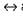as two way implication.

Detailed Solution for Test: Mathematical Logic- 2 - Question 1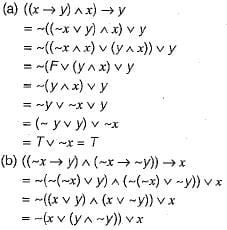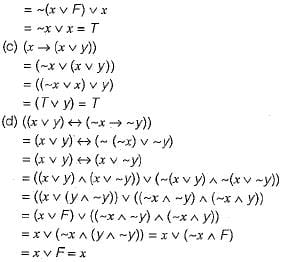Test: Mathematical Logic- 2 - Question 2

### What is the converse of the following assertion? I stay only if you go

Detailed Solution for Test: Mathematical Logic- 2 - Question 2

Let p : l stay
q ; you go
I stay only if you go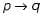Converse of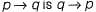Now convert the answers one-by-one into boolean form. Only option (a) i.e. “1 stay if you go” converts to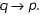Test: Mathematical Logic- 2 - Question 3

### Consider two well-formed formulas in propositional logic: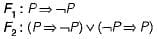Which of the following statements is correct?

Detailed Solution for Test: Mathematical Logic- 2 - Question 3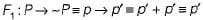So F1 is contingency. Hence, F1 is satisfiable but not valid.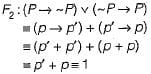So F2 is tautology and therefore valid.

Test: Mathematical Logic- 2 - Question 4

“If X then Y unless Z” is represented by which of the following formulas in propostional logic? ("_") is negation, “∧” is conjunction, and "→" is implication)

Detailed Solution for Test: Mathematical Logic- 2 - Question 4

If X then Y unless Z is represented by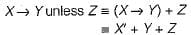Now convert the answers one-by-one into boolean form only choice (a) converts to X'+ Y + Z as can be seen below: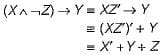Test: Mathematical Logic- 2 - Question 5

Let P, Q and, R be three atomic propositional assertions. Let X denote ( P v Q ) → R and Y denote (P → R) v (Q → R). Which one of the following is a tautology?

Detailed Solution for Test: Mathematical Logic- 2 - Question 5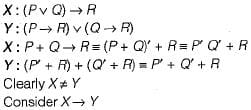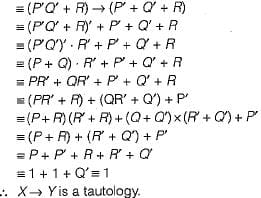Test: Mathematical Logic- 2 - Question 6

Which one of the following is the most appropriate logical formula to represent the statement:
“Gold and silver ornaments are precious”
The following notations are used:
G(x): x is a gold ornament
S(x): x is a silver ornament
P(x): x is precious

Detailed Solution for Test: Mathematical Logic- 2 - Question 6

The correct translation of "Gold and silver ornaments are precious ” is choice (d)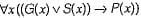which is read as “if an ornament is gold or silver, then it is precious”.
Now since a given ornament cannot be both gold and silver at the same time.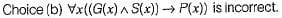Test: Mathematical Logic- 2 - Question 7

Suppose the predicate P(x, y, t) is used to represent the statement that person x can fool person y at time t. Which one of the statements below expresses best the meaning of the formula​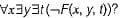Detailed Solution for Test: Mathematical Logic- 2 - Question 7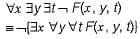≡  it is not true that (someone can fool all people at all time)
≡  no one can fool everyone all the time

Test: Mathematical Logic- 2 - Question 8

What is the logical translation of the following statements?
“None of my friends are perfect"

Detailed Solution for Test: Mathematical Logic- 2 - Question 8

None of my friends are perfect i.e., all of my friends are not perfect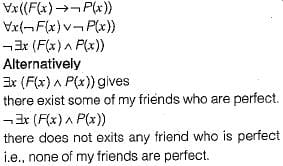Test: Mathematical Logic- 2 - Question 9

Consider the statement:
"Not all that glitters is gold"
Predicate glitters (x) is true if x glitters and predicate gold (x) is true if x is gold. Which one of the following logical formulae represents the above statement?

Detailed Solution for Test: Mathematical Logic- 2 - Question 9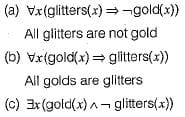There exist gold which is not glitter i.e. not all golds are glitters.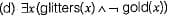Not all that glitters is gold i.e., there exist some which glitters and which is not gold.

Test: Mathematical Logic- 2 - Question 10

Which one of the following propositional logic formulas is TRUE when exactly two of p, q, and r are TRUE?

Detailed Solution for Test: Mathematical Logic- 2 - Question 10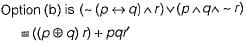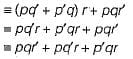This is exactly the min-term form of a logical formula which is true when exactly two variables are true (only p, q true or only p, r true or only q, r true).

Test: Mathematical Logic- 2 - Question 11

Which one of the following Boolean expressions is NOT a tautology?

Detailed Solution for Test: Mathematical Logic- 2 - Question 11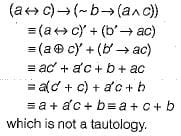Test: Mathematical Logic- 2 - Question 12

Consider the following statements:
P : Good mobile phones are not cheap
Q : Cheap mobile phones are not good
L : P implies Q
M : Q implies P
N : P is equivalent to Q
Which one of the following about L, M and N is CORRECT?

Detailed Solution for Test: Mathematical Logic- 2 - Question 12

g : mobile is good
c : mobile is cheap
P : Good mobile .phones are not cheap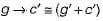Q : Cheap mobile phones are not good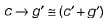∴ Both P and Q are equivalent.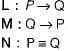Since both P and Q are equivalent, all three of L, M, N are true.

Test: Mathematical Logic- 2 - Question 13

Consider the following two statements:
S1: If a candidate is known to be corrupt, then he will not be elected.
S2: If a candidate is kind, he will be elected.
Which one of the following statements follows from S1 and S2 as per sound inference rules of logic?

Detailed Solution for Test: Mathematical Logic- 2 - Question 13

C : Person is corrupt
K : Person is kind.
E : Person is elected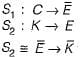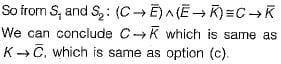## GATE Electrical Engineering (EE) 2023 Mock Test Series

22 docs|274 tests
 Use Code STAYHOME200 and get INR 200 additional OFF Use Coupon Code
Information about Test: Mathematical Logic- 2 Page
In this test you can find the Exam questions for Test: Mathematical Logic- 2 solved & explained in the simplest way possible. Besides giving Questions and answers for Test: Mathematical Logic- 2, EduRev gives you an ample number of Online tests for practice

## GATE Electrical Engineering (EE) 2023 Mock Test Series

22 docs|274 tests